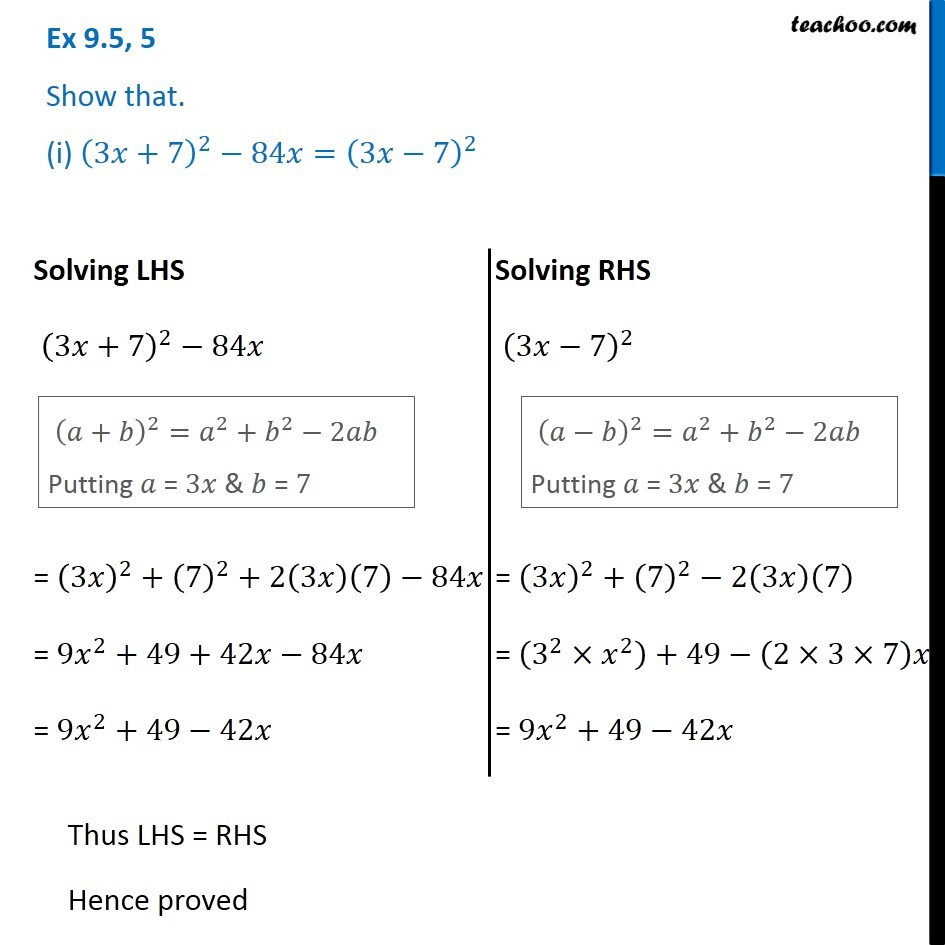Algebra Identities - Identity III

Chapter 8 Class 8 Algebraic Expressions and Identities
Concept wiseLearn in your speed, with individual attention - Teachoo Maths 1-on-1 Class

### Transcript

Question 5 Show that. (i) (3𝑥+7)^2−84𝑥=(3𝑥−7)^2 Solving LHS (3𝑥+7)^2−84𝑥 = (3𝑥)^2+(7)^2+ 2(3𝑥)(7)−84𝑥 = 9𝑥^2+49+42𝑥−84𝑥 = 9𝑥^2+49−42𝑥 (𝑎+𝑏)^2=𝑎^2+𝑏^2−2𝑎𝑏 Putting 𝑎 = 3𝑥 & 𝑏 = 7 = (3𝑥)^2+(7)^2+ 2(3𝑥)(7)−84𝑥 = 9𝑥^2+49+42𝑥−84𝑥 = 9𝑥^2+49−42𝑥 Solving RHS (3𝑥−7)^2 (𝑎−𝑏)^2=𝑎^2+𝑏^2−2𝑎𝑏 Putting 𝑎 = 3𝑥 & 𝑏 = 7 = (3𝑥)^2+(7)^2−2(3𝑥)(7) = (3^2×𝑥^2 )+49−(2×3×7)𝑥 = 9𝑥^2+49−42𝑥 Thus LHS = RHS Hence proved